14 – Determination – U(2) & SU(2)

 < ρℝεν | ℂσητεητs | ℕεχτ >“There exists, if I am not mistaken, an entire world which is the totality of mathematical truths, to which we have access only with our mind, just as a world of physical reality exists, the one like the other independent of ourselves, both of divine creation.” – Charles Hermite

In the last Chapter, we defined the Unitary Group of degree n, or U(n), to be the set of n × n Unitary Matrices under multiplication (as well as explaining what made a matrix Unitary, i.e. its Conjugate Transpose also being its inverse). We also spent time constructing the smallest Unitary Group, U(1). This was something that we did in two different ways, one geometrically inspired involving the Complex Plane and one using the more typical definition relating to Complex Matrices (though of course, in the case of U(1), we looked at 1 × 1 Complex Matrices, which are just Complex Numbers). Here we move on to look at U(2), a Group for which the matrix approach is the most appropriate one to adopt.

One motivation for considering U(2) here is that its Subgroup, SU(2), is one of the Groups featured in the Standard Model. We will meet SU(2) in the second half of this Chapter and define it in terms of restrictions on members of U(2).

2 × 2 is more Complex…Based on the general characterisation of Unitary Groups that we established in the previous Chapter, we can define the Unitary Group of degree 2, U(2), as follows:Where a, b, … , g, h ∈ ℝ.

Or alternatively:Where w, x, y, z ∈ ℂ.

It may be established that a generic member of the 2 × 2 unitary matrices, U(2), takes the following form: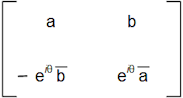Where a and b ∈ ℂ, θ is an angle with 0 ≤ θ < 2π and |a|2 + |b|2 = 1.

Demonstrating this is going to take a little effort, so if the reader would like to save themselves the trouble, then the following box can be skipped and we will meet again on the other side.

 The Shape of Things U(2) ComeIn order to corroborate the general form of a member of U(2) that we stated above, I’ll need to lay some groundwork. First of all, we have already implicitly used different ways to represent Complex Numbers. The first has been relative to the Complex Plane, where a given Complex Number, z, is equal to a + ib, where a is its coordinate on the Real (x) axis and b is its coordinate on the Imaginary (y) axis. The second, which so far has been restricted to Complex Numbers appearing on a unit circle centred at the origin, has related to drawing a line from the origin (0 + i0) to a point z in the Complex Plane, which defines an angle, θ between this line and the x-axis running East from the origin. A diagram demonstrating this construction appears at the beginning of this Chapter. By definition, a Complex Number lying on the unit circle will be a distance of 1 from the origin. This simplifies both Trigonometry and Pythagoras’s Theroem by making one of the input values equal to one. However we can adapt the definition we have used to cover any Complex Number using the following diagram:If a generic Complex Number z is given by a + ib, then the shortest distance between z and the origin is the length of the hypotenuse of the triangle shown above. Using our |x| notation, which means “size of x” then, when we employ Pythagoras, we see that: |z| = |a + ib| = √(a2 + b2) Then, using a similar approach to what we did with roots of unity, we can then say that: z = |z| cos θ + i |z| sin θ Where θ is the angle that z makes with the positive x-axis. Using Euler’s Formula we can also say that: z = |z| eiθ It may be deduced that, by following this approach, z can be wholly defined by the angle it makes with the x-axis, θ, and the length of the line from the origin to the point z, i.e |z| . The second preliminary observation relates to multiplying a number by its complex conjugate. If z = a + ib is a complex number then readers will recall that its conjugate is z = a – ib. We then have: z z = (a + ib)(a – ib) = a2 – iab + iab – (ib)2 Cancelling the positive and negative terms and recalling of course that i = -1, we get: z z = a2 + b2 = |z|2 The above argument also of course shows that: z z = a2 + b2 = |z|2 We will use both of the above in what follows. Let’s now consider a matrix A with entries {a, b}{c, d}, recalling that a, b, c, d ∈ ℂ. If this is a Unitary Matrix then we know that AAH = AHA = 1. Which written out longhand means we can form two matrix equations, first of all: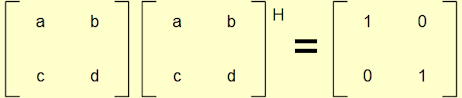Which we can write more explicitly as: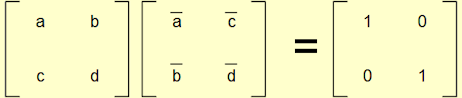From the matrix equation, we can derive the following four linear equations: a a + b b = 1      (1) a c + b d = 0      (2) c a + d b = 0 c c + d d = 1      (3) I have labelled three of these as we will be using them later. Second, we also have: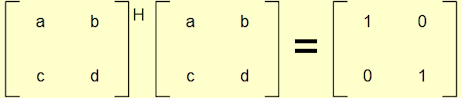Which again we can write more explicitly as:Which gives us a second set of linear equations as follows: a a + c c = 1      (4) a b + c d = 0 b a + d c = 0 b b + d d = 1      (5) Comparing (1) and (4) we see that: a a + b b = 1 a a + c c = 1 Which leads us to deduce that: b b = c c thus: c = b b / c Equally if we now also consider (1) and (5), we have: a a + b b = 1 b b + d d = 1 Which leads us to deduce that: d d = a a thus: d = a a / d So we can write our generic matrix as follows:Considering (2) we have: a c + b d = 0 Which we can rearrange to say that: -a c = b d Rearranging further we can see that: -a / d = b / c These are precisely elements appearing in our latest version of the generic 2 × 2 Unitary Matrix. So we can now write:What else can we tell about a / d? Well looking at (3) and (4) we have: c c + d d = 1 a a + c c = 1 By our second preliminary observation, we can write these as: |c|2 + |d|2 = 1 |a|2 + |c|2 = 1 Subtracting the former from the latter we get: |a|2 + |c|2 – |c|2 – |d|2 = 1 – 1 Which gives us: |a|2 – |d|2 = 0 or |a|2 = |d|2 At the beginning of this box we showed that |z| = |z|. We can use this fact to also state: |a|2 = |d|2 Which we can write as: |a|2 / |d|2 = 1 Which, noting that |x| is always a positive Real Number (ruling out -1 as a solution), means that: |a| / |d| = 1 and also that: |a / d| = 1 This final result means that a / d is a Complex Number whose length from the origin is restricted to be 1. But this is just another way of saying that a / d lies on the unit circle. As we saw in Chapter 11, we can describe any such number as being equal to eiθ for some angle θ. If we substitute eiθ for a / d in our matrix we can write:Which is of course the formulation of a 2 × 2 Unitary Matrix that we wanted to establish. Again leveraging (1) we can add the further restriction that: |a|2 + |b|2 = 1

U(2) Can be a GroupHaving established a generic matrix to represent members of U(2), we can use this finding to demonstrate that this set is indeed a Group. Before doing so, it is worth noting three facts about complex conjugates. First if we consider two complex numbers x and y then:

(xy) = x y

I.e. the conjugate of the result of a multiplication is the same as multiplying and then conjugating.

 To demonstrate this long-hand: (a + bi)(c + di) = ac + adi + bci + bdi2 = ac – bd + (ad + bc)i      (6) Whereas: (a – bi)(c – di) = ac – adi – bci + bdi2 = ac – bd – (ad + bc)i      (7) By definition (7) is the conjugate of (6).

Second if we consider what the term eiθ means, we should recall that this is a complex number located a length of 1 from the origin and at an angle to the Real axis of θ. The Real axis mirror that we used to explain complex conjugation in Chapter 7 then means that (this time using “*” to denote the complex conjugate for the aforementioned typographical reasons):

(eiθ)* = ei(-θ)

I.e. a complex number 1 away from the origin and at an angle of -θ to the Real axis.

Third, the conjugate of a number that has been conjugated is the original number. So (returning to the bar notation that I’ll employ whenever I can):

a + ib = a – ib

and

a – ib = a + ib

which gets us back to where we started.

We can combine these three facts in one equality as follows (again using the asterisk notation):

(eiθ a* b)* = ei(-θ) a b*

Precisely this type of result will come in in handy very shortly.

Having established these three facts about conjugation, let’s see whether or not U(2) is indeed a Group.

1. Closure

If we take two matrices A and B belonging to U(2), both will have the same general format, which we can write as:If we multiply these together, we get: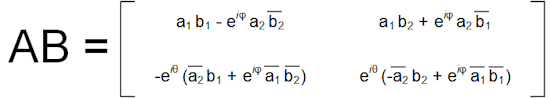This result looks almost like our canonical form for a member of U(2), but we can see that the term eiφ seems to be associated with the wrong pairings of as and bs. Also, eiφ should be replaced with its complex conjugate, ei(-φ). We can address both of these issues, by multiplying the terms outside of both the brackets in the lower row by eiφ and dividing the terms within the brackets in the same row by eiφ . Here we can also recall from the properties of powers that:

ex / ey = e(x – y)

and (as a specific case of the above when x = 0, noting that – as referenced before – anything to the power zero is equal to 1):

1 / ey = e(-y)

So this means our trickery with eiφ leads to the following matrix:It may be seen that this matrix is in the standard format for a member of U(2) and so U(2) is closed under matrix multiplication.

2. Identity

The regular 2 × 2 identity serves this role. It can be seen that this is a member of U(2) by setting a = 1, b = 0 and θ = 0 in our canonical format for a member of U(2).

3. Inverses

We know that – by definition – the inverse of any Unitary Matrix is its conjugate transpose. But – given that if we take the conjugate transpose of a conjugate transpose we end up with the original matrix – we can see that the conjugate transpose of a Unitary Matrix must itself be unitary.

4. Associative

Matrix multiplication is associative, so this holds.

So we have established that U(2) is also a Group.

In a box in Chapter 13, we established that U(1) ≅ SO(2) (i.e. the two Groups are isomorphic). While we cannot easily extend this relationship for U(2), a further property of this Group is also worth noting. In Chapter 6, we met Orthogonal Matrices which related to reflections and rotations of 2 dimensional space and whose entries were Real Numbers. It can be shown (though I don’t propose to do this here) that any 2 × 2 Unitary Matrix U can be expressed as an Orthogonal Matrix sandwiched between two Complex Diagonal Matrices. This looks something like:This equality demonstrates an underlying kinship between Unitary Matrices (with entries in Complex Numbers) and Orthogonal Matrices (with entries in Real Numbers).

With these findings we have come to the end of our introductory review of Unitary Groups. Unlike U(1), U(2) does not play a role in the standard model, however its Subgroup SU(2) and SU(2)’s bigger relative, SU(3) [a Subgroup of U(3)], both do. The time we have spent looking at Unitary Groups will inform our understanding of Special Unitary Groups, a subject to which we will turn our attention in the next section.

Singular DeterminationIn Chapter 13, we made the following two definitions:

A Unitary Matrix is a matrix M such that its Conjugate Transpose is its inverse. That is:

MMH = MHM = 1

and

The Unitary Group of degree n, denoted by U(n), is the set of all n × n Unitary Matrices under matrix multiplication.

Our next step is to move from the Unitary Groups, U(n), to the Special Unitary Groups, SU(n). The latter are defined by placing a further restriction on the former, namely:

The Special Unitary Group of degree n, denoted by SU(n), is the set of all n × n Unitary Matrices, with a determinant of 1, under matrix multiplication.

This definition immediately implies that SU(n) ⊂ U(n), but actually SU(n) is also a Subgroup of U(n), something we will prove for n=2 below.

SU(2) a Worked Example

Using our definition above when n=2, we can see that SU(2) is the set of 2 × 2 unitary matrices which also have a determinant of 1. We can write this as follows:Where w, x, y, z ∈ ℂ and det(A) means the determinant of matrix A.

As mentioned above, given that U(2) is the Group of all 2 × 2 unitary matrices, we can immediately see that SU(2) is at least a subset of U(2) . This also means that any member of SU(2) is also of the same form as members of U(2), i.e.:Where a and b ∈ ℂ, θ is an angle with 0 ≤ θ < 2π and |a|2 + |b|2 = 1.

To the above, we now need to add the further requirement that the determinant is equal to 1. We have previously seen that the determinant of a matrix is an important concept and we established some results relating to this for the Orthogonal Group of degree 2, O(2) in Chapter 6. Using our canonical definition of a member of U(2) and recalling that for a generic 2 × 2 matrix {a, b}{c, d} its determinant is given by ad – bc, we can note that the determinant of any member of U(2) is equal to:

a a eiθ + b b eiθ =

eiθ(a a + b b)

But (using one of the properties of complex conjugates we referenced above) we can write this as:

eiθ(|a|2 +|b|2)

But a restriction of matrices in U(2) is that |a|2 +|b|2 = 1. So the determinant of any element of U(2) is precisely eiθ.

So, if we want to move from members of U(2) to those of SU(2), then we need to set eiθ to 1. This means that a generic member of SU(2) is just:Where a and b ∈ ℂ and and |a|2 + |b|2 = 1.

In Chapter 8, we showed that the requirements for a subset of a Group to be a Subgroup were as follows:

Given a Group (G,*), a set H ⊂ G is a Subgroup (H,*) if the following holds:

1. For every a and b ∈ H, a * b ∈ H

2. For every a ∈ H, a-1 ∈ H

Let’s apply this criteria to SU(2) within U(2).

1. Closure

If we take two elements of SU(2) in the canonical form and multiply them, we get the following:So this requirement is satisfied .

2. Inverses

First recall from Chapter 5 that the inverse of a 2 × 2 matrix is given by:If we apply this to our general format for a member of SU(2), we get:We also know that the determinant of any matrix A ∈ SU(2) is – by definition – equal to 1. So we can simplify this to:The matrix on the right is in the canonical form and so it is a member of SU(2) and we have inverses .

So SU(2) is indeed a Subgroup of U(2), which of course also proves that SU(2) is a Group in its own right.

Back in Chapter 7, we met a number system called the quaternions, I then alluded to a relationship between these and a Group we would meet later. Having just met the Group, namely SU(2), the following box details this linkage.

 A Study in Quartet If we think about a generic element of SU(2), we have shown above that this is:Where a and b ∈ ℂ and |a|2 + |b|2 = 1. In an approach which we will come back to when considering Vector Spaces in Chapter 16, it is relatively easy to see that if a matrix, M is part of SU(2), then we can write M as: M = aE + bI + cJ + dK Where E is the identity matrix, I, J and K are members of SU(2) which are shown below  and a, b, c and d are Real Numbers.With little effort, it is possible to see that these matrices can be mapped to the basic elements of the quaternions (1, i, j, and k) and will satisfy all of the required relationships: i2 = j2 = k2 = ijk = – 1. Indeed the Group SU(2) is isomorphic to the Group formed by the quaternions, another one of those linkages that we will continue to come across as we progress on our journey.

We will come back to look at some of the properties of SU(2) in later chapters, before upping the ante by looking at SU(3). However, having established both that SU(2) is a Group and shown what a generic elements of SU(2) looks like, we are going to have to take time to introduce some more Mathematical apparatus before we can resume our journey towards SU(3) × SU(2) × U(1). The next Chapter and the one that follows it will introduce some new concepts and new structures relating to space; space in the Mathematical sense that is. We will return to our perennial topic of matrices in Chapter 17 (not for the last time). Then, in the following three Chapters, starting from Chapter 18, we will build on this start, by considering the work of the Mathematician Sophus Lie and thereby bring things back to Group Theory; learning some useful things about linkages to Particle Physics along the way. Having laid some substantial foundations, Chapter 21 will see us pick up from where this Chapter has left off, by exploring what SU(3) actually looks like.

 Concepts Introduced in this Chapter Determinant of Unitary Matrices The determinant of any 2 × 2 Unitary Matrix can be expressed as eiθ, for some angle θ. I.e. these determinants lie on the unit circle. Diagonalisation of Unitary Matrices Any Unitary Matrix, U, can be expressed in terms of two Complex Diagonal Matrices D1 and D2 and an Orthogonal (which implies Real) Matrix O. That is: U = D1 O D2
 Groups Discovered in this Chapter U(2) The Group of 2 × 2 Unitary Matrices under multiplication. A general element of U(2) looks like this:Where a and b ∈ ℂ, θ is an angle with 0 ≤ θ < 2π and |a|2 + |b|2 = 1. SU(n) The Special Unitary Group of degree n, denoted by SU(n), is the set of all n × n Unitary Matrices, with a determinant of 1, under matrix multiplication. This definition immediately implies that SU(n) ⊂ U(n). In fact SU(n) < U(n). SU(2) The Special Unitary Group of degree 2. The Group of 2 × 2 Unitary Matrices, with a determinant of 1, under multiplication. A general element of this is a 2 × 2 matrix with the following entries:Where a and b ∈ ℂ and |a|2 + |b|2 = 1.
 < ρℝεν | ℂσητεητs | ℕεχτ >

Chapter 14 – Notes

  This approach points to a shift of our coordinate frame from a rectangular to a polar one, but this is not something that we need to explore further at this juncture.  Clearly if we multiply an expression by something and then divide it by the same something then the value of the expression is not changed, i.e.: a x / a = x  We will consider whether or not it is a Subgroup soon enough.  We can also prove closure of SU(2) in another way, by leveraging the following property of determinanats (which I won’t prove here): For any two n × n matrices A and B: det(AB) = det(A) det(B) In particular, if both det(A) and det(B) are equal to 1, then so is det(AB). Because U(2) is closed and SU(2) ⊂ U(2), this means that the product of two members of SU(2) is already known to be in U(2). We have now shown that such a product’s determinant is equal to 1. A member of U(2) with a determinant of 1 is a member of SU(2) by definition, so SU(2) is closed. It should be noted that this proof also applies to the more general case; it demonstrates that SU(n) is closed, not just SU(2).  As with closure of SU(2) we can make this argument in a different way, one that again relies upon the properties of determinants, namely that: For an n × n matrix, A: det(AH) = det(A) For any matrix A ∈ SU(2), we also have A ∈ U(2). This means that A-1 ∈ U(2) as well (because U(2) is a Group). We also know that for any A ∈ U(2), A-1 = AH. But, by the property we have introduced above: det(AH) = det(A) but also det(A) = 1 as A ∈ SU(2) which leads to the observation that: det(A) = 1 = 1 as the conjugate of a Real Number is the same number. If we combine these two equalities, we see that: det(AH) = det(A) = 1 which implies that AH ∈ SU(2), so A-1 ∈ SU(2), establishing the required result. As with the previous footnote, the above argument has the virtue of holding for all Groups SU(n), not just SU(2).  We will also meet these matrices again in Chapter 19 where they form the basis of a particular type of Vector Space.
 Text: © Peter James Thomas 2016-17. Images: © Peter James Thomas 2016-17, unless stated otherwise. Published under a Creative Commons Attribution 4.0 International License.